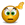# VALUE!

## Excel Help for Value! in Analyzing Corporate Financial DataI am doing calculations on a spread sheet and it has come up wiyth the error Value, I want it to be blank so when I add the column up is does it correctly.I am trying to multiply 2 columns, one is a quantity and the is a result of an if  and lookup function. Do I multiply thje two columns or do I need to repeat how I got the calculations. Posted by freddo on 17 Jul 2012Rate this: (3/5 from 1 vote) To catch formula errors and return alternative values you can use the ISERROR function to catch any errors and replace the VALUE! with a blank or any other value such as zero.Support your formula is A1/A2, then the formula would be:=IF(ISERROR(A1/A2),"",A1/A2) Excel Business Forums Administrator Posted by Excel Helper on 17 Jul 2012Rate this: (4/5 from 3 votes) To multiply more than two columns in Excel, you can use the multiplication formulas, but include several cells or ranges.For example, to multiply values in columns B, C and D, use one of the following formulas:Multiplication operator: =A2*B2*C2PRODUCT function: =PRODUCT(A2:C2)Array formula (Ctrl + Shift + Enter): =A2:A5*B2:B5*C2:C5 Let me know if this helps.Regards,Jerry M. Jerry M Posted by jerry_maguire on 21 Dec 2020